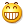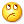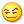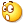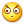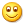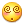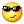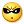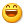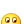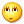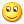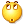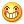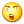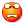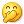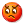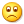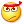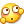<转载>PHP 数组使用之道
in LaravelPHP with 0 comment## 入门

``````<?php
\$keys = ['sky', 'grass', 'orange'];
\$values = ['blue', 'green', 'orange'];

\$array = array_combine(\$keys, \$values);

print_r(\$array);
// Array
// (
//     [sky] => blue
//     [grass] => green
//     [orange] => orange
// )``````

``````<?php

print_r(array_keys(\$array));// ['sky', 'grass', 'orange']

print_r(array_values(\$array));// ['blue', 'green', 'orange']

print_r(array_flip(\$array));
// Array
// (
//     [blue] => sky
//     [green] => grass
//     [orange] => orange
// )``````

## 简化代码

list() 函数，确切的说它不是一个函数，而是一种语言结构，可以在单次操作中将数组中的值赋值给一组变量。举个例子，下面给出 list() 函数的基本使用：

``````<?php
// 定义数组
\$array = ['a', 'b', 'c'];

// 不使用 list()
\$a = \$array;
\$b = \$array;
\$c = \$array;

// 使用 list() 函数
list(\$a, \$b, \$c) = \$array;``````

``````\$string = 'hello|wild|world';

list(\$hello, , \$world) = explode('|', \$string);
echo \$hello, ' ', \$world;``````

``````\$arrays = [[1, 2], [3, 4], [5, 6]];

foreach (\$arrays as list(\$a, \$b)) {
\$c = \$a + \$b;

echo \$c, ', ';
}``````

``````<?php
\$array = [
'clothes' => 't-shirt',
'size' => 'medium',
'color' => 'blue',
];

extract(\$array);

echo \$clothes, ' ', \$size, ' ', \$color;``````

extract() 函数的逆操作是 compact() 函数，用于通过变量名创建关联数组：

``````<?php
\$clothes = 't-shirt';
\$size = 'medium';
\$color = 'blue';

\$array = compact('clothes', 'size', 'color');
print_r(\$array);

// Array
// (
//     [clothes] => t-shirt
//     [size] => medium
//     [color] => blue
// )``````

## 过滤函数

PHP 提供一个用于过滤数组的超赞的函数，它是 array_filter()。将待处理数组作为函数的第一个参数，第二个参数是一个匿名函数。如果你希望数组中的元素通过验证则在匿名函数返回 true，否则返回 false

``````<?php

\$numbers = [20, -3, 50, -99, 55];

\$positive = array_filter(\$numbers, function (\$number) {
return \$number > 0;
});

print_r(\$positive);// [0 => 20, 2 => 50, 4 => 55]``````

``````<?php
\$numbers = [-1, 0, 1];

\$not_empty = array_filter(\$numbers);

print_r(\$not_empty);// [0 => -1, 2 => 1]``````

``````<?php
\$array = [1, 1, 1, 1, 2, 2, 2, 3, 4, 5, 5];

\$uniques = array_unique(\$array);

print_r(\$uniques);
print_r(\$array);
// Array
// (
//      => 1
//      => 2
//      => 3
//      => 4
//      => 5
// )``````

array_column() 函数可以从多维数组（multi-dimensional）中获取指定列的值，如从 SQL 数据库中获取答案或者 CSV 文件导入数据。只需要传入数组和指定的列名：

``````<?php
\$array = [
['id' => 1, 'title' => 'tree'],
['id' => 2, 'title' => 'sun'],
['id' => 3, 'title' => 'cloud'],
];

\$ids = array_column(\$array, 'id');

print_r(\$ids);// [1, 2, 3]``````

``````<?php
\$cinemas = Cinema::find()->all();
\$cinema_ids = array_column(\$cinemas, 'id'); // php7 forever!``````

## 数组遍历处理

``````<?php
\$cities = ['Berlin', 'KYIV', 'Amsterdam', 'Riga'];
\$aliases = array_map('strtolower', \$cities);

print_r(\$aliases);// ['berlin', 'kyiv, 'amsterdam', 'riga']

\$numbers = [1, -2, 3, -4, 5];
\$squares = array_map(function (\$number) {
return \$number ** 2;
}, \$numbers);

print_r(\$squares);// [1, 4, 9, 16, 25]``````

``````<?php
\$model = ['id' => 7, 'name' => 'James'];
\$res = array_map(function (\$key, \$value) {
return \$key . ' is ' . \$value;
}, array_keys(\$model), \$model);

print_r(\$res);
// Array
// (
//      => id is 7
//      => name is James
// )``````

``````<?php
\$fruits = [
'banana' => 'yellow',
'apple' => 'green',
'orange' => 'orange',
];

array_walk(\$fruits, function (&\$value, \$key) {
\$value = \$key . ' is ' . \$value;
});

print_r(\$fruits);``````

## 数组连接操作

``````<?php
\$array1 = ['a' => 'a', 'b' => 'b', 'c' => 'c'];
\$array2 = ['a' => 'A', 'b' => 'B', 'D' => 'D'];

\$merge = array_merge(\$array1, \$array2);
print_r(\$merge);
// Array
// (
//     [a] => A
//     [b] => B
//     [c] => c
//     [D] => D
// )``````

``````<?php
\$array1 = [1, 2, 3, 4];
\$array2 = [3, 4, 5, 6];

\$diff = array_diff(\$array1, \$array2);
\$intersect = array_intersect(\$array1, \$array2);

print_r(\$diff); // 差集 [0 => 1, 1 => 2]
print_r(\$intersect); //交集 [2 => 3, 3 => 4]``````

## 数组的数学运算

``````<?php

\$numbers = [1, 2, 3, 4, 5];

print_r(array_sum(\$numbers));// 15

print_r(array_product(\$numbers));// 120

print_r(array_reduce(\$numbers, function (\$carry, \$item) {
return \$carry ? \$carry / \$item : 1;
}));// 0.0083 = 1/2/3/4/5``````

``````<?php

\$things = ['apple', 'apple', 'banana', 'tree', 'tree', 'tree'];
\$values = array_count_values(\$things);

print_r(\$values);

// Array
// (
//     [apple] => 2
//     [banana] => 1
//     [tree] => 3
// )``````

## 生成数组

``````<?php
\$bind = array_fill(0, 5, '?');
print_r(\$bind);``````

``````<?php
\$letters = range('a', 'z');
print_r(\$letters); // ['a', 'b', ..., 'z']

\$hours = range(0, 23);
print_r(\$hours); // [0, 1, 2, ..., 23]``````

``````<?php
\$numbers = range(1, 10);
\$top = array_slice(\$numbers, 0, 3);

print_r(\$top);// [1, 2, 3]``````

## 排序数组

• a 保持索引关系进行排序
• k 依据键名排序
• r 对数组进行逆向排序
• u 使用用户自定义排序规则排序

akru
aasort arsortuasort
k ksortkrsort
rarsortkrsortrsort
uuasort usort

## 数组函数的组合使用

``````<?php
\$values = ['say', '  bye', '', ' to', ' spaces  ', '    '];
\$words = array_filter(array_map('trim', \$values));

print_r(\$words);// ['say', 'bye', 'to', 'spaces']``````

``````<?php
\$models = [\$model, \$model, \$model];

\$id_to_title = array_combine(
array_column(\$models, 'id'),
array_column(\$models, 'title')
);

print_r(\$id_to_title);``````

``````<?php

\$letters = ['a', 'a', 'a', 'a', 'b', 'b', 'c', 'd', 'd', 'd', 'd', 'd'];

\$values = array_count_values(\$letters);
arsort(\$values);
\$top = array_slice(\$values, 0, 3);

print_r(\$top);``````

``````<?php
\$order = [
['product_id' => 1, 'price' => 99, 'count' => 1],
['product_id' => 2, 'price' => 50, 'count' => 2],
['product_id' => 2, 'price' => 17, 'count' => 3],
];

\$sum = array_sum(array_map(function (\$product_row) {
return \$product_row['price'] * \$product_row['count'];
}, \$order));

print_r(\$sum);// 250``````

## 原文

Working With PHP Arrays in the Right Way

Responses